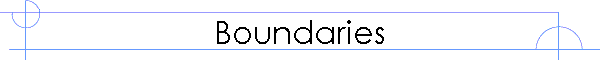Ultrasonics Applications and Solutions for Industry and Research

## Propagation of a Sound Wave Through an Interface Barrier

What happens to a sound wave when it interacts with some type of discontinuity?  Each material has some particular acoustic impedance.  This acoustic impedance is given by the following equation: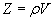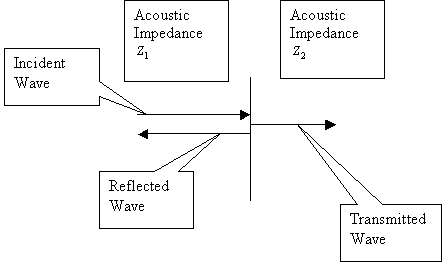Where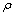is the density and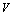is the speed of sound.  Consider a sound wave propagating between two different materials.  The first material has acoustic impedance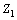and the second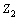.  For simplicity let's assume the wave arrives perpendicular to the surface.  Some of the sound wave will propagate through the boundary and some will be reflected.

A sound wave that reaches an interface between two materials will generate a reflected wave and a transmitted wave.  The Transmission coefficient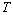measures the fraction of energy that passes through the barrier and the reflection coefficient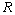measures the fraction of energy reflected back.

Physically we might expect that the difference between the acoustic impedance values of the two materials might have something to do with how much sound is transmitted and how much is reflected.  Suppose that we could adjustto be any value we wanted.  If we make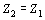then there should be no reflection at all and the transmitted wave should be exactly the same as the incident wave.  Ifis much larger or smaller thanthen most of the energy would be reflected at the boundary.

It's also reasonable to expect that the total amplitude of in the Incident, Reflected and Transmitted waves must remain constant.  Since all the waves meet at a thin layer, regardless of what happens on either side, the sound waves must smoothly join together.  In other words, we expect the following to hold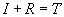It turns out that the reflection coefficient and the transmission coefficient can be written down as follows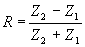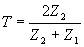Notice that if we set, then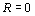and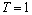.

If we have a wave that reaches a boundary and the wave has an amplitude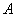then the amplitudes of the reflected and transmitted waves are given by the following equations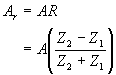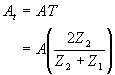Note that the amplitude of the reflected wave can be positive or negative.  A negative value corresponds to a 180 degree phase change.  In other words, the wave will appear upside down with respect to the incident wave.

The "coupling efficiency" between a transducer and a material is actually the transmission coefficient.  In order to calculate this coupling efficiency it's necessary to know the acoustic impedance of the transducer and the material.  If the acoustic impedance of the material is not known, then we can't calculate the transmission coefficient.

Home ] Up ]